### Home > CALC > Chapter 11 > Lesson 11.2.3 > Problem11-71

11-71.
1.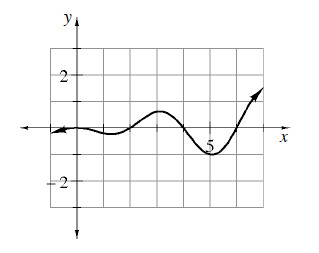Multiple Choice: The graph below represents f ′(x). If f(0) = 5, then this is the value of: Homework Help ✎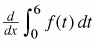1. 0

2. 5

3.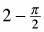4.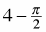5.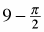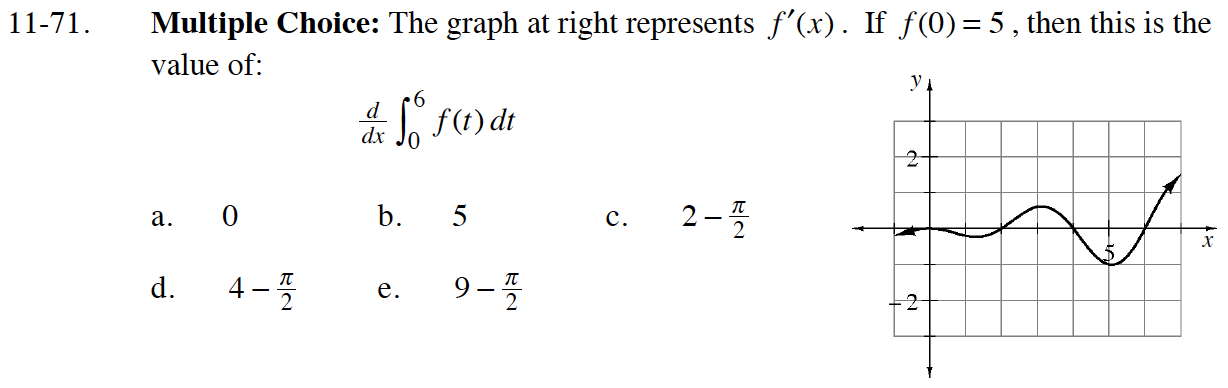If you evaluate the integral, do you get a function or a constant? What is the derivative of this function/constant?